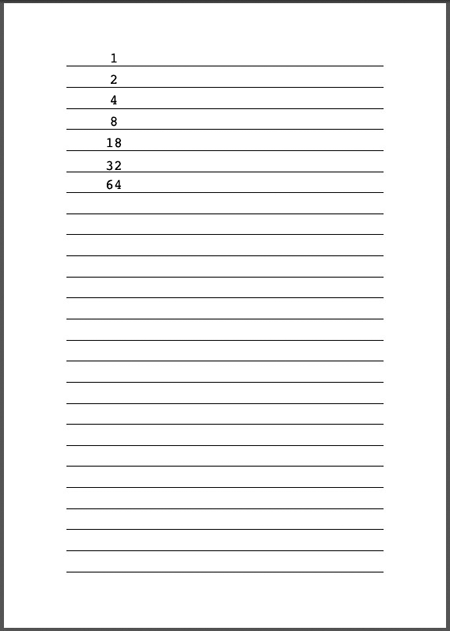SEARCH HOMEMath Central Quandaries & QueriesQuestion from Donald: If I save a penny a week,double it every week. How many weeks will it take to reach 1,000,000 dollarsHi Donald,

You are working with pennies and there are 100 pennies in a dollar so I suggest you look for how many weeks it will take to reach 100,000,000 cents.

Do an experiment to get a feeling for how fact the number of pennies grows. Get a sheet of lined pages similar to the one belowTreat the lines on the page as weeks and on each line write how many pennies you have that week. 1 on the first week, 2 on the second week and so on. There are probably less than 30 lines on the page so it won't take long.

If you want to approach the problem algebraically you need to solve $2^n = 10,000,000$ for $n.$ The standard way to solve this is to use logarithms.

I hope this helps,
PennyMath Central is supported by the University of Regina and The Pacific Institute for the Mathematical Sciences.